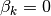# statsmodels.discrete.discrete_model.Logit.fit_regularized¶

Logit.fit_regularized(start_params=None, method='l1', maxiter='defined_by_method', full_output=1, disp=1, callback=None, alpha=0, trim_mode='auto', auto_trim_tol=0.01, size_trim_tol=0.0001, qc_tol=0.03, **kwargs)

Fit the model using a regularized maximum likelihood. The regularization method AND the solver used is determined by the argument method.

Parameters: start_params : array-like, optional Initial guess of the solution for the loglikelihood maximization. The default is an array of zeros. method : ‘l1’ or ‘l1_cvxopt_cp’ See notes for details. maxiter : Integer or ‘defined_by_method’ Maximum number of iterations to perform. If ‘defined_by_method’, then use method defaults (see notes). full_output : bool Set to True to have all available output in the Results object’s mle_retvals attribute. The output is dependent on the solver. See LikelihoodModelResults notes section for more information. disp : bool Set to True to print convergence messages. fargs : tuple Extra arguments passed to the likelihood function, i.e., loglike(x,*args) callback : callable callback(xk) Called after each iteration, as callback(xk), where xk is the current parameter vector. retall : bool Set to True to return list of solutions at each iteration. Available in Results object’s mle_retvals attribute. alpha : non-negative scalar or numpy array (same size as parameters) The weight multiplying the l1 penalty term trim_mode : ‘auto, ‘size’, or ‘off’ If not ‘off’, trim (set to zero) parameters that would have been zero if the solver reached the theoretical minimum. If ‘auto’, trim params using the Theory above. If ‘size’, trim params if they have very small absolute value size_trim_tol : float or ‘auto’ (default = ‘auto’) For use when trim_mode == ‘size’ auto_trim_tol : float For sue when trim_mode == ‘auto’. Use qc_tol : float Print warning and don’t allow auto trim when (ii) (above) is violated by this much. qc_verbose : Boolean If true, print out a full QC report upon failure

Notes

Extra parameters are not penalized if alpha is given as a scalar. An example is the shape parameter in NegativeBinomial nb1 and nb2.

Optional arguments for the solvers (available in Results.mle_settings):

'l1'
acc : float (default 1e-6)
Requested accuracy as used by slsqp
'l1_cvxopt_cp'
abstol : float
absolute accuracy (default: 1e-7).
reltol : float
relative accuracy (default: 1e-6).
feastol : float
tolerance for feasibility conditions (default: 1e-7).
refinement : int
number of iterative refinement steps when solving KKT
equations (default: 1).


Optimization methodology

With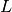the negative log likelihood, we solve the convex but non-smooth problem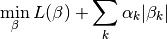via the transformation to the smooth, convex, constrained problem in twice as many variables (adding the “added variables”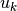)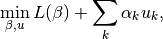subject to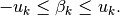With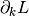the derivative ofin the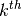parameter direction, theory dictates that, at the minimum, exactly one of two conditions holds:

1.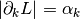and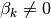2.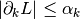and